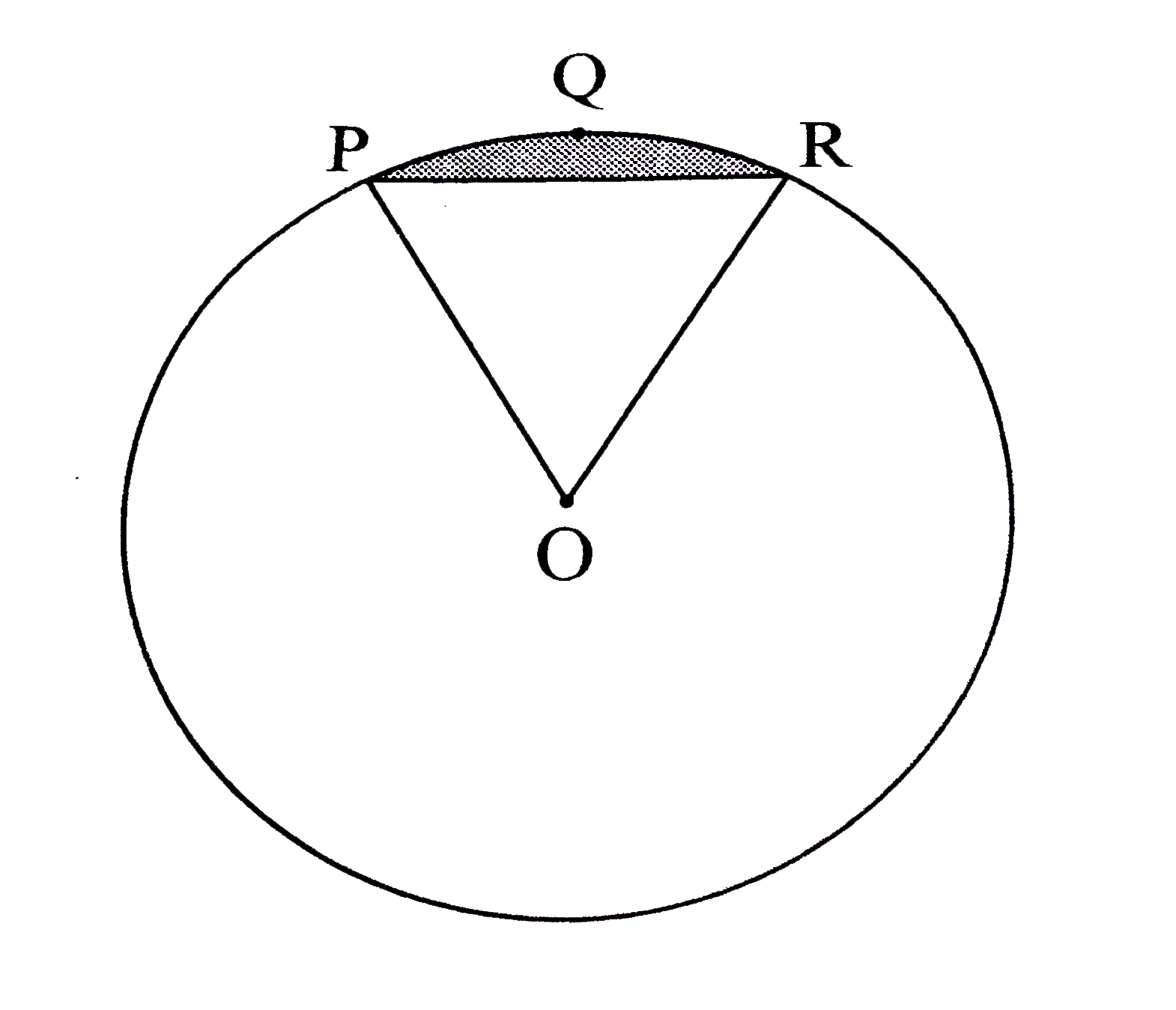Doubtnut App - Get 50 Lakh+ video solutions better than GoogleInstall Now

# In the figure, O is the centre of the circle. m(arc PQR)=60∘ OP = 10 cm. Find the area of the shaded region. (π=3.14,√3=1.73)Text Solution
Verified by Experts

## The radius of the circle ( r) = OP = 10 cm m(arc PQR)=θ=60∘ The shaded region is segment PQR. A(segment PQR) =r2[πθ360−sinθ2] =102[3.14×60360−sin602] =100[3.146−√32×2]...[∵sin60=√32] 100×[3.146−1.734]...(∵√3=1.73) =100[3.14×2−1.73×312] =100[6.28−5.1912] =100×1.0912=10912 =9.083≈9.08cm2